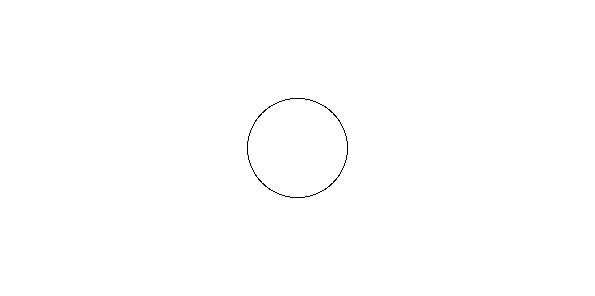Related Articles

# Plot a circle using centre point and radius in MATLAB

• Last Updated : 08 Apr, 2019

The aim is to plot a circle using center point and radius in MATLAB without using inbuilt functions for plotting. A black and white image can be represented as a 2 order matrix. The first order is for the rows and the second order is for the columns, the pixel value will determine the color of the pixel based on the grayscale color format.

Approach :

• We are given with a point and radius. Let the coordinates of the centre point be (x1, y1) and radius be R.
• We find the distance of centre point to every pixel(i, j)th.
`dist = sqrt((j-c)^2+(i-r)^2);`
• Now if dist=R i.e radius we make that pixel black.

Below is the implementation:

 `% MATLAB code to plot circle using centre and radius.`` ` `% create a white image of size 300X600``I=zeros(300, 600)+1;`` ` `% Radius of circle``R=50;`` ` `% coordinates of centre point``c=300;``r=150;`` ` `% accessing every pixel``for` `i=1:size(I, 1)``    ``for` `j=1:size(I, 2)``        ``dist=round(sqrt((j-c)^2+(i-r)^2));``        ``if` `dist==R``            ``I(i, j)=0;``        ``end``    ``end``end`` ` `% display the image``figure, imshow(I);`

Output :My Personal Notes arrow_drop_up